# Power analysis

Dewesoft Power is one of the most complex mathematical modules inside Dewesoft modules which provide all functions which are needed for an analysis of power grids.

Some Dewesoft power module advantages are:

• When measuring voltage and current there are several ways to calculate the formula. Even with knowing basic equations, real world measurement is by far not that simple. Transducers and amplifiers have different transfer characteristics, which needs to be corrected, lots of AD cards have also phase shifts between channels and the line frequency is not ideal but varies with time. Power module compensates all possible errors and is checked to be perfect tool for power measurements even for most demanding applications.

• Dewesoft power module can not only handle the line to earth voltages but also the line to line voltages. Depending on the selected type of wiring schematic the conversion is done inside.

• In addition to the phasors also the RMS values of voltage and current, the phase angles and the power values can be shown for different power systems.

• Based on a calculation in the frequency domain the input channels can be calibrated very efficient in amplitude as well as in phase. Internal amplifiers, external transformers and clamps can be corrected with the use of this function.

• The range of applications for the systems is very wide:

• $P$, $Q$, $S$, $D$
• $\cos\phi$, Power factor
• $P$, $Q$, $\cos\phi$ for each harmonic
• Symmetrical Components (positive, negative and zero sequence components)
• Period values (½ cycle, cycle,…)
• All frequencies can be analyzed. In addition to harmonics FFT a full frequency based FFT is available.

• If more then one power module is defined each one can have it‘s own frequency. So it is possible to do power measurements on different frequency systems with only one instrument at the same time.

To measure Power, we need at least two input channels connected to your instrument for measuring voltage and current.

• Required hardware - Dewesoft Sirius
• Required software - SE or higher + POWER option or EE
• Setup sample rate - At least 5 kHz

For additional help with Power module visit Dewesoft PRO training → Dewesoft Web pagePRO Training.

## Power module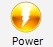After adding the Power module and opening it by clicking on icon, following setup screen is opened: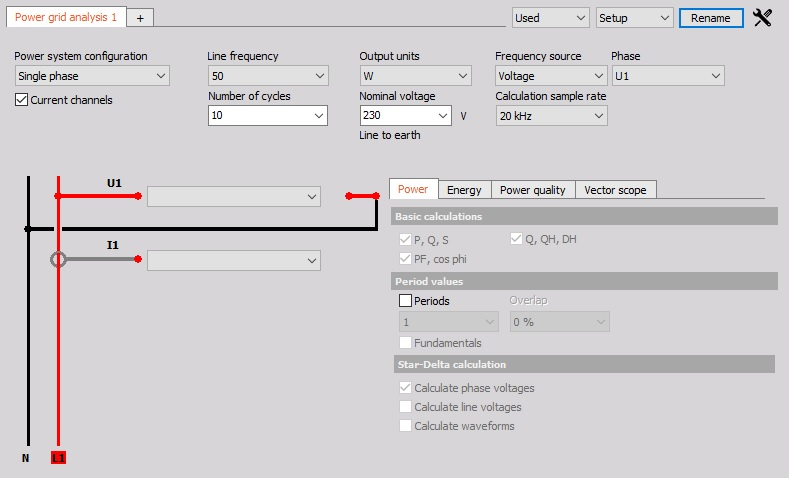For detailed information on how to initialize Power module see → Add module.

Power grid analysis setup is divided in three main categories:

• Configuration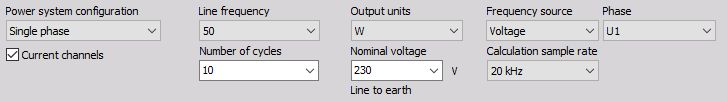• Wiring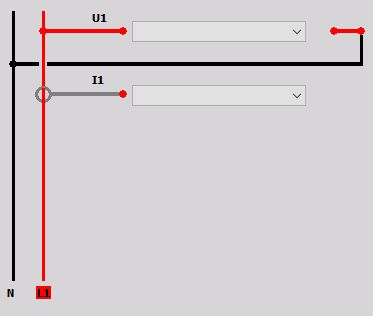• Calculations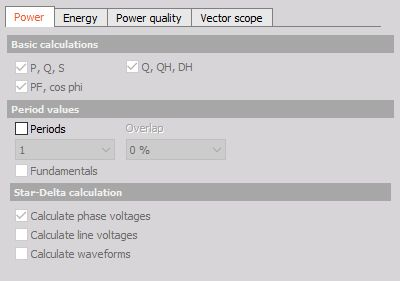NOTE: We need to have the correct unit for voltage and current. Voltage units could be V, kV and MV while the current units could be either A or kA. These values we entered in the Units field.

### Voltage measurements

We can split voltage measurements into several sections: high voltage grid measurements (in kilovolts), where we need voltage transducers, direct low voltage grid measurements (120/230 V) and low voltage measurements (up to 50 V). The high voltage converters convert kilovolts voltage signals to a measurable range - up to one kilovolt.

It is very important that the measurement Range is chosen according to the expected signal. We need to take special care for the settings of the Low-pass filter. If this setting is lower than half of the sample rate, it will cut the signals already in the range of the measurements. Sometimes this is needed, but more often this filter is set by mistake to the low range and then the measurement results will be invalid.

### Current measurements

Basic procedures of Power application setup are:

• Power module setup for applied hardware
• Calibration of current

For additional information visit: Dewesoft WEB Power Manual